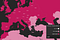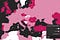# Ok, neighborhood analysis is important, how do I do it in a web GIS application?

`CountryObject = {    ISO: "",    Name: "",    Value: "",    Neighbors: []}countriesLoop:for (var c = 0; c < countries.length; c++) {    var neighbors = [];neighborsLoop:    for (var n = 0; n < neighborsTable.length; n++) {        if (neighborsTable[n].fromCountryISO === countries[c].ISO) {            neighbors.push(neighborsTable[n])            if (neighbors.length === 4) {                countries[c].neighbors = neighbors;                break neighborsLoop;            }        }    }}`
`If (neighborsTable[n].distance < thresholdDistance) { … }`

# Time for spatial calculationsSpatial Moving Average of Covid-19 new cases per millions of population (Data as of 31 Dec 2020).Spatial Similarity Index of Covid-19 new cases per millions of population (Data as of 31 Dec 2020).
`countriesLoop:for (var c = 0; c < countries.length; c++) {neighborsLoop:for (var n = 0; n < countries[c].neighbors.length; n++) {var SumNeighborsValue = countries[c].neighbors[n].Value++}var meanNeighborsValue = SumNeighborsValue / countries[c].neighbors.lengthcountries[c].SMAValue = (SumNeighborsValue + countries[c].Value) / countries[c].neighbors.length + 1 // Spatial Moving Average, the sum of neighbors' values plus current spatial entity’s value to the number of neighbors plus the current spatial entitycountries[c].SSIValue = countries[c].Value / meanNeighborsValue // Spatial Similarity Index, the current spatial entity’s value to the mean value of neighbors}`

PhD candidate and freelance developer

## More from George Panagiotopoulos

PhD candidate and freelance developer